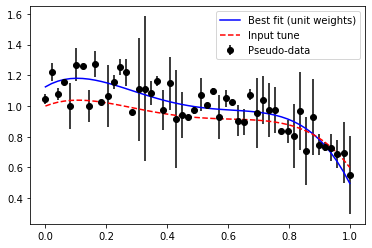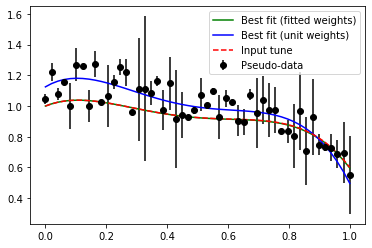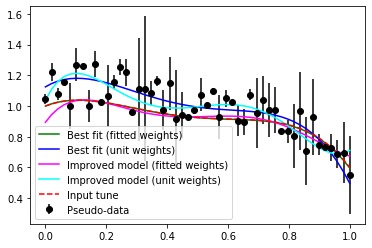# test

In this post - which is really a jupyter notebook - I illustrate a cute principle of relevant for tuning of event generators. Under most circumstances, tuning refers to the process of fitting a large number of generator parameters to data. In that process, not all data should be weighted equally, and data points are assigned weights depending on how important their reproduction is deemed.

Consider now the reverse problem. We have a tune, which someone made at some point in the past, with weights unknown. Maybe they just guessed. It describes our data to some degree. We make a slight improvement of the model, and we now want to figure out if the "improvement" really makes things better, compared to the old one. It depends of course on our choice of weights. Since we don't know what weights were initially used, we have to try and re-estimate them. We therefore start out by fitting the weights, using the original tune as the target, and then make our comparison with the same weights.

In this constructed example we end up in a situation where this procedure renders our improvement worse than the original guess. Go figure.

In :
import numpy as np
import matplotlib.pyplot as plt

np.random.seed(12)

# First generate some pseudo-data. This corresponds to real data from an experiment.
nd = 50 # Number of data points
x = np.linspace(0, 1, nd)
y = np.cos(x) + 0.3*np.random.rand(nd)
# Gaussian errors
errorsize = 0.15
yerr = abs(np.random.normal(0., errorsize, nd))

# Use a degree n polynomial with some given coefficients as our initial "tune"
degree = 4 # polynomial degree
coeffs = [-4.8, 8.6, -5.0, 0.8, 1.0]
#coeffs = [0.4, -1.4,  0.7, 1.0, 1.1]
# Our tune is then this
tune = np.poly1d(coeffs)

# And the "true" best fit in contrast.
w = [1 for i in range(0,nd)]
f = np.polyfit(x, y, degree, w=[ww * 1./np.sqrt(ye) for ww,ye in zip(w,yerr)])
p = np.poly1d(f)
print("Best fit (unit weights) coefficients = ")
print(f)

# Plot the stuff.
xplot = np.linspace(0, 1, 200)
plt.errorbar(x, y, yerr=yerr, fmt='o',color='black',label="Pseudo-data")
plt.plot(x,p(x),color='blue',label="Best fit (unit weights)")
plt.plot(x,tune(x),'--',color='red',label="Input tune")

plt.legend()
plt.show()

Best fit (unit weights) coefficients =
[-7.25749584 13.08124948 -7.65191241  1.19961955  1.1249718 ]In :
# We now want to figure out which weights we should use in the fit to reproduce "coeffs".
# That is: I want to get "coeffs" from the frame above as the result when I run np.polyfit.
import scipy.optimize as opt

# A chi2 function, taking the weights as an input
def chi2(weights):
testCoeffs,cov = np.polyfit(x,y,degree,cov=True,w=[ww * 1./np.sqrt(ye) for ww,ye in zip(weights,yerr)])
errs = np.diagonal(cov)
chi2 = 0.
for testC, targetC, err in zip(testCoeffs,coeffs, errs):
chi2 += (targetC - testC)**2/err
return chi2/float(degree)

# Here lies a challenge! If the initial guess on the weights
# is far away from the target, the fit will have a hard time converging.
# Maybe we can do better than standard minimization techniques. Genetic algorithm, maybe?
w0 = w
fittedWeights =  opt.minimize(chi2, w0, tol=1e-6)
# Let's take a look at the fitted weights
print("Fitted weights = ")
print(fittedWeights.x)

# And repeat the original fit with the new weights.
fOpt = np.polyfit(x, y, degree, w=[ww * 1./np.sqrt(ye) for ww,ye in zip(fittedWeights.x,yerr)])
pOpt = np.poly1d(fOpt)

print("Best fit (fitted weights) coefficients = ")
print(fOpt)
print("Best fit (unit weights) coefficients = ")
print(f)
print('The original "tune" = ')
print(coeffs)

# And plot the lot together
plt.errorbar(x, y, yerr=yerr, fmt='o',color='black',label="Pseudo-data")
plt.plot(x,pOpt(x),'-',color='green',label="Best fit (fitted weights)")
plt.plot(x,p(x),color='blue',label="Best fit (unit weights)")
plt.plot(x,tune(x),'--',color='red',label="Input tune")

plt.legend()
plt.show()

Fitted weights =
[ 0.15155217 -0.25691222 -0.17584135  0.00747454  2.83798671 -0.65697859
-0.27964302  3.35625859 -0.55634395  5.02668368  0.7494724  -0.57752997
-0.94712285  0.11174089 -1.17571161  0.85882534  0.92843806  0.68819698
0.72071715  1.10796187  1.10567054  1.16917514  1.18392499  6.96993834
0.9757265   0.88198121 -0.23092779  0.95111715  1.20191562  0.77307405
0.11674472  1.5217488   1.75152796  0.67514409  1.03896673  1.0293936
0.99271696  0.98071387  1.25216692  1.00512878  0.97155575  1.20116812
0.93560342  1.49075797  0.54202057  0.38192639  1.23878003  1.03355266
1.31524601  0.25413592]
Best fit (fitted weights) coefficients =
[-4.80040606  8.59790455 -5.00145934  0.79981842  0.99999778]
Best fit (unit weights) coefficients =
[-7.25749584 13.08124948 -7.65191241  1.19961955  1.1249718 ]
The original "tune" =
[-4.8, 8.6, -5.0, 0.8, 1.0]In :
# Now we add a "better" model, and see if it improves the overall description of data.

# Get a global chi2 model vs. data, possibly weighted
def globalChi2(dataX, dataY, yerrs, model, weights=[]):
if len(weights) ==0:
weights = [1 for i in range(0,len(dataX))]
mVals = [model(xx) for xx in dataX]
chi2 = 0.
sow = 0.
for mv, target, te, w in zip(mVals, dataY, yerrs, weights):
chi2 += w*(target - mv)**2/np.sqrt(te)
sow+=w*np.sqrt(te)
return chi2

w = [1 for i in range(0,nd)]
# First with unit weights
fImprovedUnit = np.polyfit(x, y, degree+1, w=[ww * 1./np.sqrt(ye) for ww,ye in zip(w,yerr)])
pImprovedUnit = np.poly1d(fImprovedUnit)
# Then with fitted weights
fImproved = np.polyfit(x, y, degree+1, w=[ww * 1./np.sqrt(ye) for ww,ye in zip(fittedWeights.x,yerr)])
pImproved = np.poly1d(fImproved)

print("Global GOF:")
print("Tune:")
print(globalChi2(x, y, yerr, tune))
print("Best fit unit weights:")
print(globalChi2(x, y, yerr, p))
print("Best fit fitted weights:")
print(globalChi2(x, y, yerr, pOpt))
print("Improved model unit weights:")
print(globalChi2(x, y, yerr, pImprovedUnit))
print("Improved model fitted weights:")
print(globalChi2(x, y, yerr, pImproved))

# And plot the lot together
plt.errorbar(x, y, yerr=yerr, fmt='o',color='black',label="Pseudo-data")
plt.plot(x,pOpt(x),'-',color='green',label="Best fit (fitted weights)")
plt.plot(x,p(x),color='blue',label="Best fit (unit weights)")
plt.plot(x,pImproved(x),'-',color='magenta',label="Improved model (fitted weights)")
plt.plot(x,pImprovedUnit(x),color='cyan',label="Improved model (unit weights)")
plt.plot(x,tune(x),'--',color='red',label="Input tune")

plt.legend()
plt.show()

Global GOF:
Tune:
3.3593707363574286
Best fit unit weights:
1.6249657437801772
Best fit fitted weights:
3.372673037202404
Improved model unit weights:
1.6045732329447893
Improved model fitted weights:
3.681641919829624In [ ]: